# Throw

We throw 2 times with 2 dices. What is the probability that the first roll will fall more than sum of 9 and the second throw have sum 3 or does not have the sum 4?

p =  55.5556 %

### Step-by-step explanation: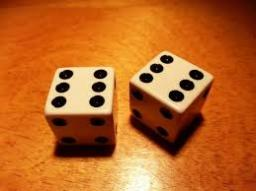Did you find an error or inaccuracy? Feel free to write us. Thank you!Tips to related online calculators
Would you like to compute count of combinations?

## Related math problems and questions:

• Fall sum or sameFind the probability that if you roll two dice, it will fall the sum of 10, or the same number will fall on both dice.
• Dices throwsWhat is the probability that the two throws of the dice: a) Six falls even once b) Six will fall at least once
• Sum or productWhat is the probability that two dice fall will have the sum 7 or product 12?
• We rollWe roll two dice A. - what is the probability that the sum of the falling numbers is at most 4 B. - is at least 10 C. - is divisible by 5?
• DiceWe throw 10 times a playing dice. What is the probability that the six will fall exactly 4 times?
• Sum of fall dices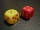What is the probability that the sum of 9 will fall on a roll of two dice? Hint: write down all the pairs that can occur as follows: 11 12 13 14 15. . 21 22 23 24. .. . 31 32. .. . . . . . .. . 66, count them, it's the variable n variable m: 36, 63,. .. .
• One threeWe throw two dice. What is the probability that max one three falls?
• DiceWe throw five times the dice. What is the probability that six fits exactly twice?
• DicesWe will throw two dice. What is the probability that the ratio between numbers on first and second dice will be 1:2?
• Sum 10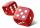What is the probability that two dice throw twice in a row will result the sum of 10?
• Two diceWe roll two dice. What is the probability that the sum of the falling numbers is greater than 3?
• Three dice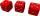What is the probability that when we roll three dice, we roll the numbers 1,2,3?
• Sum on diceWe have two dice. What is the greater likelihood of fall a total sum 7 or 8 ? (write 7, 8 or 0 if the probabilities are the same)?
• Six on diceWhat is the probability that when throwing two dice will fall at least one six?
• Dice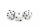How many times must throw the dice, the probability of throwing at least one six was greater than 90%?
• The diceWhat is the probability of events that if we throw a dice is rolled less than 6?
• A jackpot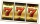How many times must I play this jackpot to win? A jackpot of seven games having (1 X 2), i. E. , home win or away win.